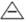Chapter 8.1, Problem 24ESMathematical Excursions (MindTap C...

4th Edition
Richard N. Aufmann + 3 others
ISBN: 9781305965584

Solutions

Chapter
SectionMathematical Excursions (MindTap C...

4th Edition
Richard N. Aufmann + 3 others
ISBN: 9781305965584
Textbook Problem

Military Time. Evaluate each expression, where + and − indicate addition and subtraction, respectively, using military time. (Military time uses a 24-hour clock, where 2:00 A.M. is equivalent to 0200 hours and 10 P.M. is equivalent to 2200 hours.) 0600 − 2200

To determine

The addition 06002200 using military time.

Explanation

Given information: denote the addition on 24-hour clock military time.

In military time, 2:00 A. M. is equivalent to 0200 hours and 10:00 P. M. is equivalent to 2200 hours.

Calculation:

0600

Still sussing out bartleby?

Check out a sample textbook solution.

See a sample solution

The Solution to Your Study Problems

Bartleby provides explanations to thousands of textbook problems written by our experts, many with advanced degrees!

Get Started

Find more solutions based on key concepts